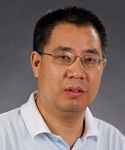1992 博士，西安交通大学

1988 硕士，西安交通大学

1985 学士，四川大学

1998年至今  教授/博士生导师，西安交通大学

2004年至2005 高级研究员，比利时Katholieke Universiteit Leuven大学

1999年至2000 客座研究员，香港城市大学

1993年至1998 副教授，西安交通大学

1996年至1997 博士后，香港中文大学

1. O. Wing, Y. L. Jiang, and Q. J. Yu, “Rational approximation of irrational functions by linear fractional transformations,” IEEE Transactions on Circuits and Systems - Part I, Vol. 45, No. 11, pp. 1216-1221, 1998
2. Y. L. Jiang and O. Wing, “Monotone waveform relaxation for systems of nonlinear differential-algebraic equations,” SIAM Journal on Numerical Analysis, Vol. 38, No. 1, pp. 170-185, 2000
3. Y. L. Jiang and O. Wing, “A note on the spectra and pseudo spectra of waveform relaxation operators for linear  differential-algebraic equations,” SIAM Journal on Numerical Analysis, Vol. 38, No. 1, pp. 186-201, 2000
4. Y. L. Jiang, R. M. M. Chen, and O. Wing, “Convergence analysis of waveform relaxation for nonlinear differential-algebraic equations of index one,” IEEE Transactions on Circuits and Systems - Part I, Vol. 47, No. 11, pp. 1639-1645, 2000
5. Y. L. Jiang, R. M. M. Chen, and O. Wing, “Improving convergence performance of relaxation-based transient analysis by matrix splitting in circuit simulation,” IEEE Transactions on Circuits and Systems - Part I, Vol. 48, No. 6, pp. 769-780, 2001
6. Y. L. Jiang, R. M. M. Chen, and O. Wing, “Waveform relaxation of nonlinear second-order differential equations,” IEEE Transactions on Circuits and Systems - Part I, Vol. 48, No. 11, pp. 1344-1347, 2001
7. Y. L. Jiang, R. M. M. Chen, and O. Wing, “Periodic waveform relaxation of nonlinear dynamic systems by quasi-linearization,” IEEE Transactions on Circuits and Systems - Part I, Vol. 50, No. 4, pp. 589-593, 2003
8. Y. L. Jiang, “On time-domain simulation of lossless transmission lines with nonlinear terminations,” SIAM Journal on Numerical Analysis, Vol. 42, No. 3, pp. 1018-1031, 2004
9. Y. L. Jiang, “A general approach to waveform relaxation solutions of differential-algebraic equations: the continuous-time and discrete-time cases,” IEEE Transactions on Circuits and Systems - Part I, Vol. 51, No. 9, pp. 1770-1780, 2004
10. Y. L. Jiang and R. M. M. Chen, “Computing periodic solutions of linear differential-algebraic equations by waveform relaxation,” Mathematics of Computation, Vol. 74, No. 250, pp. 781-804, 2005
11. G. J. Peng, Y. L. Jiang, and C. P. Li, “Bifurcations of a Holling-type II predator-prey system with constant rate harvesting,” International Journal of Bifurcation and Chaos, Vol. 19, No. 8, pp. 2499-2514, 2009
12. G. J. Peng and Y. L. Jiang, “Two routes to chaos in the fractional Lorenz system with dimension continuously varying,” Physical A, Vol. 389, No. 19, pp. 4140-4148, 2010
13. Y. M. Kang and Y. L. Jiang, “Spectral density of fluctuations in fractional bistable Klein-Kramers systems,” Physical Review E, Vol. 81, No. 2, pp. 021109(6p), 2010
14. Y. L. Jiang and H. B. Chen, “Application of general orthogonal polynomials to fast simulation of nonlinear descriptor systems through piecewise-linear approximation,” IEEE Transactions on Computer-Aided Design of Integrated Circuits and Systems, Vol. 31, No. 5, pp. 804-808, 2012
15. Y. L. Jiang and H. B. Chen, “Time domain model order reduction of general orthogonal polynomials for linear input-output systems,” IEEE Transactions on Automatic Control, Vol. 57, No. 2, pp. 330-343, 2012
16. M. J. Gander, Y. L. Jiang, B. Song, and H. Zhang, “Analysis of two parareal algorithms for time-periodic problems,” SIAM Journal on Scientific Computing, Vol. 35, No. 5, pp. A2393-A2415, 2013

17. Y. L. Jiang and X. Kong, “On the uniqueness and perturbation to the best rank-one approximation of a tensor,” SIAM Journal on Matrix Analysis and Applications, Vol. 36, No. 2, pp. 775-792, 2015